# Stoichiometry the calculation of quantities in chemical equations

• Slides: 17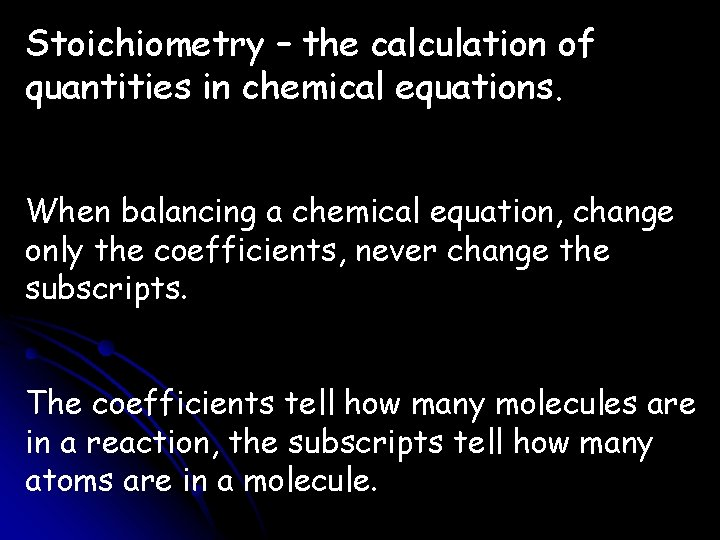Stoichiometry – the calculation of quantities in chemical equations. When balancing a chemical equation, change only the coefficients, never change the subscripts. The coefficients tell how many molecules are in a reaction, the subscripts tell how many atoms are in a molecule.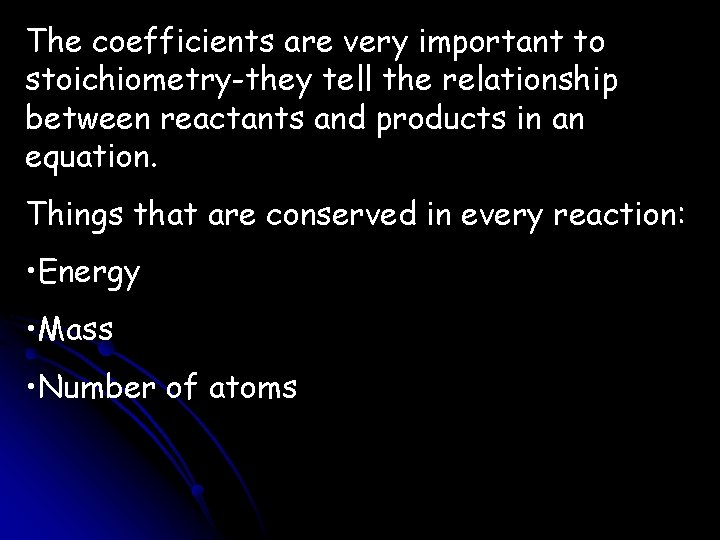The coefficients are very important to stoichiometry-they tell the relationship between reactants and products in an equation. Things that are conserved in every reaction: • Energy • Mass • Number of atoms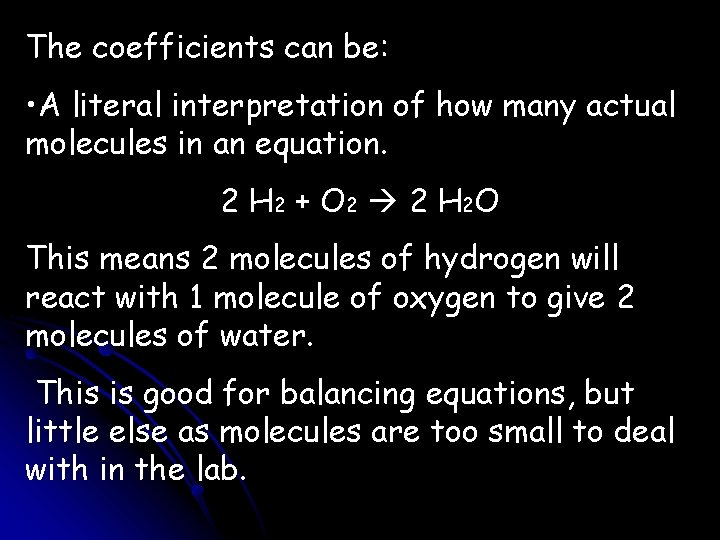The coefficients can be: • A literal interpretation of how many actual molecules in an equation. 2 H 2 + O 2 2 H 2 O This means 2 molecules of hydrogen will react with 1 molecule of oxygen to give 2 molecules of water. This is good for balancing equations, but little else as molecules are too small to deal with in the lab.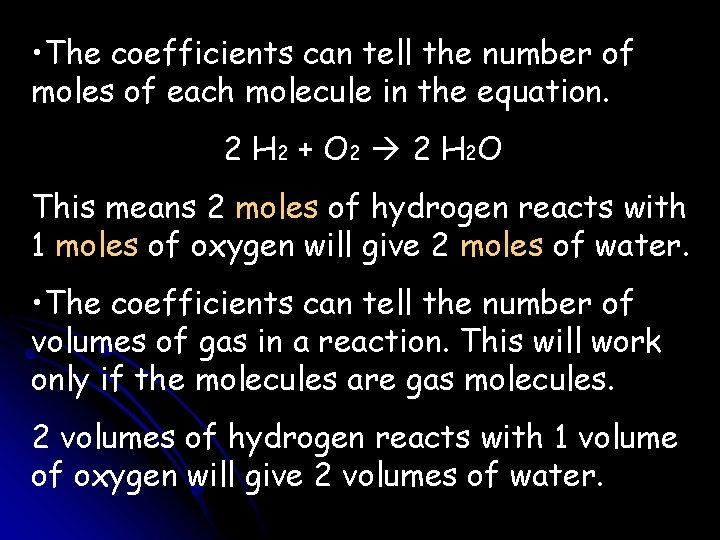• The coefficients can tell the number of moles of each molecule in the equation. 2 H 2 + O 2 2 H 2 O This means 2 moles of hydrogen reacts with 1 moles of oxygen will give 2 moles of water. • The coefficients can tell the number of volumes of gas in a reaction. This will work only if the molecules are gas molecules. 2 volumes of hydrogen reacts with 1 volume of oxygen will give 2 volumes of water.Moles Ratios – a fraction, in moles, of 2 different molecules in a balanced equation (a conversion factor for unit analysis). 2 H 2 + O 2 2 H 2 O There are three equivalencies (comparisons): 2 mol H 2 ≈ 1 mol O 2 2 mol H 2 ≈ 2 mol H 2 O 1 mol O 2 ≈ 2 mol H 2 O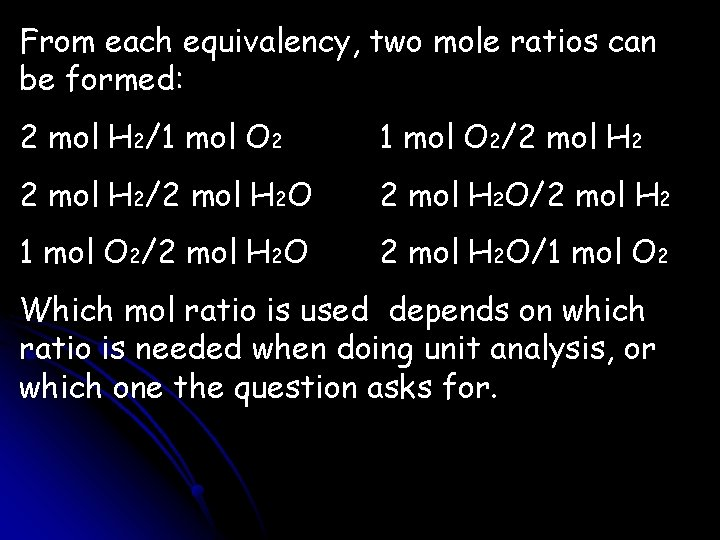From each equivalency, two mole ratios can be formed: 2 mol H 2/1 mol O 2/2 mol H 2/2 mol H 2 O/2 mol H 2 1 mol O 2/2 mol H 2 O/1 mol O 2 Which mol ratio is used depends on which ratio is needed when doing unit analysis, or which one the question asks for.N 2 + H 2 NH 3 If you start with 3. 0 moles of N 2, how many moles of H 2 are needed? How many moles of NH 3 can be produced? 1. Balance the reaction: N 2 + 3 H 2 2 NH 3 2. Write out the equivalencies: 1 mol N 2≈3 mol H 2; 1 mol N 2≈2 mol NH 3; 3 mol H 2≈2 NH 3 3. Set up the unit analysis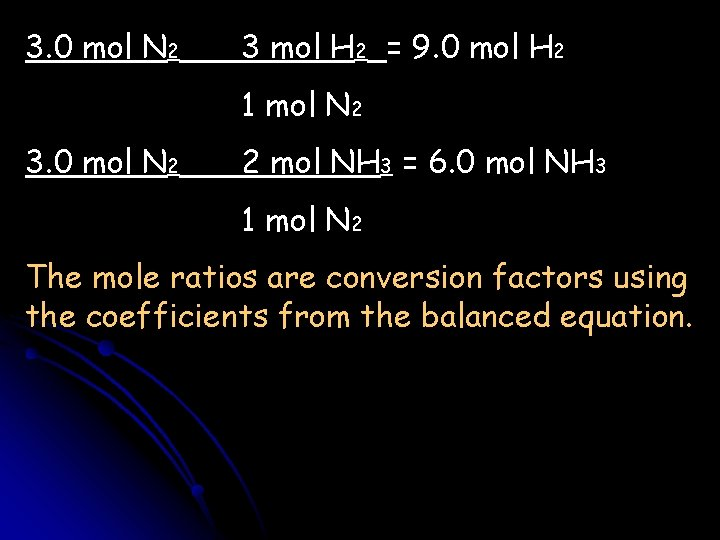3. 0 mol N 2 3 mol H 2 = 9. 0 mol H 2 1 mol N 2 3. 0 mol N 2 2 mol NH 3 = 6. 0 mol NH 3 1 mol N 2 The mole ratios are conversion factors using the coefficients from the balanced equation.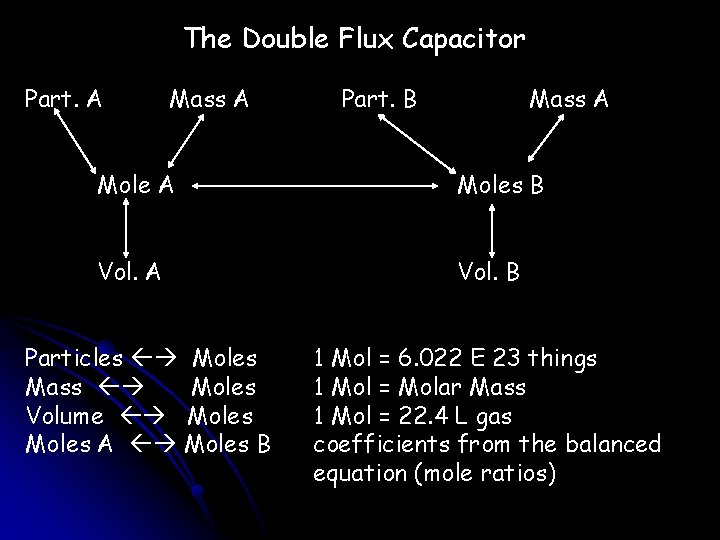The Double Flux Capacitor Part. A Mass A Part. B Mass A Moles B Vol. A Vol. B Particles Moles Mass Moles Volume Moles A Moles B 1 Mol = 6. 022 E 23 things 1 Mol = Molar Mass 1 Mol = 22. 4 L gas coefficients from the balanced equation (mole ratios)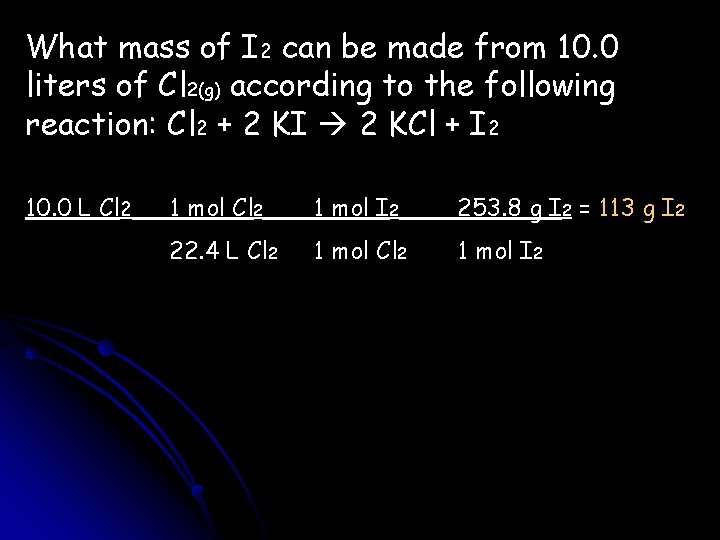What mass of I 2 can be made from 10. 0 liters of Cl 2(g) according to the following reaction: Cl 2 + 2 KI 2 KCl + I 2 10. 0 L Cl 2 1 mol I 2 253. 8 g I 2 = 113 g I 2 22. 4 L Cl 2 1 mol I 2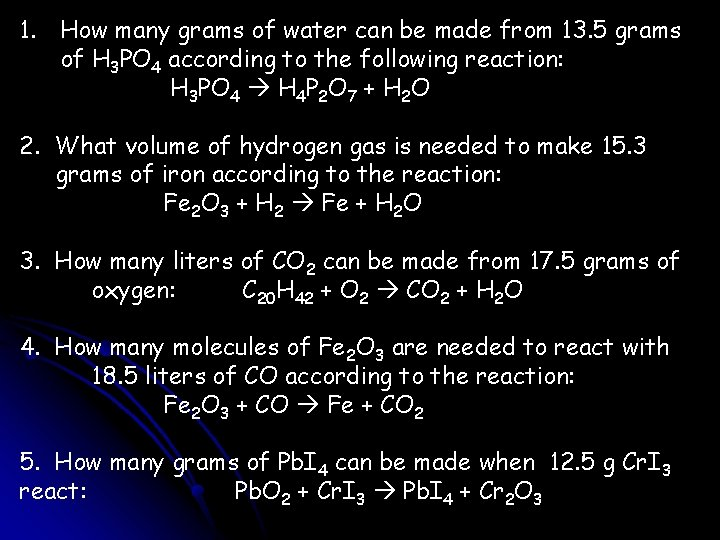1. How many grams of water can be made from 13. 5 grams of H 3 PO 4 according to the following reaction: H 3 PO 4 H 4 P 2 O 7 + H 2 O 2. What volume of hydrogen gas is needed to make 15. 3 grams of iron according to the reaction: Fe 2 O 3 + H 2 Fe + H 2 O 3. How many liters of CO 2 can be made from 17. 5 grams of oxygen: C 20 H 42 + O 2 CO 2 + H 2 O 4. How many molecules of Fe 2 O 3 are needed to react with 18. 5 liters of CO according to the reaction: Fe 2 O 3 + CO Fe + CO 2 5. How many grams of Pb. I 4 can be made when 12. 5 g Cr. I 3 react: Pb. O 2 + Cr. I 3 Pb. I 4 + Cr 2 O 3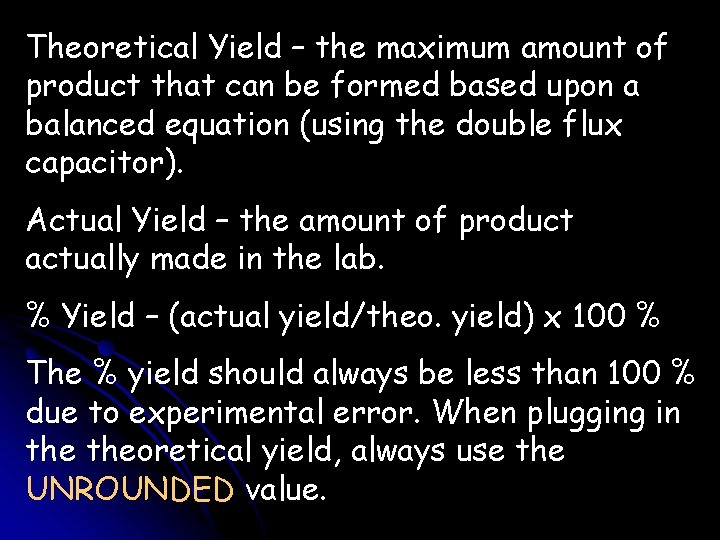Theoretical Yield – the maximum amount of product that can be formed based upon a balanced equation (using the double flux capacitor). Actual Yield – the amount of product actually made in the lab. % Yield – (actual yield/theo. yield) x 100 % The % yield should always be less than 100 % due to experimental error. When plugging in theoretical yield, always use the UNROUNDED value.The actual yield will always be one of the products. It can never be one of the reactants. Na + H 2 O Na. OH + H 2 If 18. 3 g of sodium can produce 8. 23 liters of hydrogen gas, what is the % yield? Balanced equation: 2, 2, 2, 1 18. 3 g Na 1 mol H 2 22. 4 l H 2 = 8. 91 l H 2 23. 0 g Na 2 mol Na 1 mol H 2 (8. 23 l H 2 / 8. 9113 … l H 2) x 100 % = 92. 4 %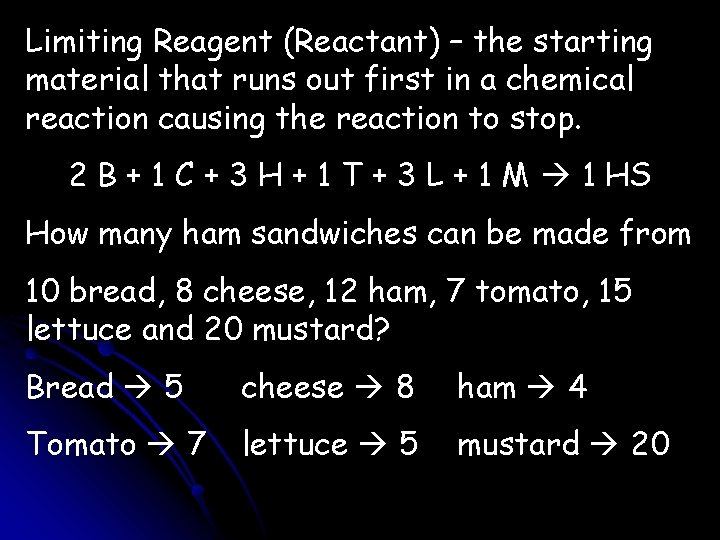Limiting Reagent (Reactant) – the starting material that runs out first in a chemical reaction causing the reaction to stop. 2 B + 1 C + 3 H + 1 T + 3 L + 1 M 1 HS How many ham sandwiches can be made from 10 bread, 8 cheese, 12 ham, 7 tomato, 15 lettuce and 20 mustard? Bread 5 cheese 8 ham 4 Tomato 7 lettuce 5 mustard 20Ham is the limiting reactant (LR) because only 4 ham sandwiches can be made. After that point, the ham runs out causing the reaction to stop. Therefore no more sandwiches can be made. When working a limiting reactant problem, work the problem twice- calculate the amount of product that can be made from each reactant. The smallest amount of product will be how much that can be made. The reactant that causes the least amount of product will be the LR.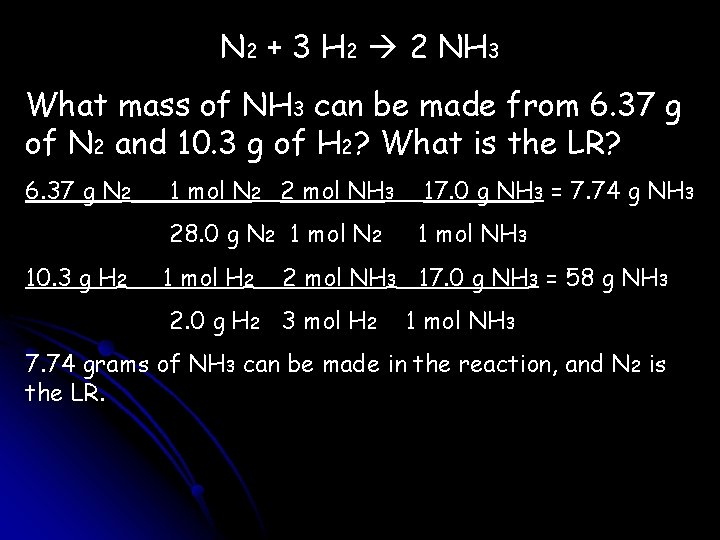N 2 + 3 H 2 2 NH 3 What mass of NH 3 can be made from 6. 37 g of N 2 and 10. 3 g of H 2? What is the LR? 6. 37 g N 2 10. 3 g H 2 1 mol N 2 2 mol NH 3 17. 0 g NH 3 = 7. 74 g NH 3 28. 0 g N 2 1 mol NH 3 1 mol H 2 2 mol NH 3 17. 0 g NH 3 = 58 g NH 3 2. 0 g H 2 3 mol H 2 1 mol NH 3 7. 74 grams of NH 3 can be made in the reaction, and N 2 is the LR.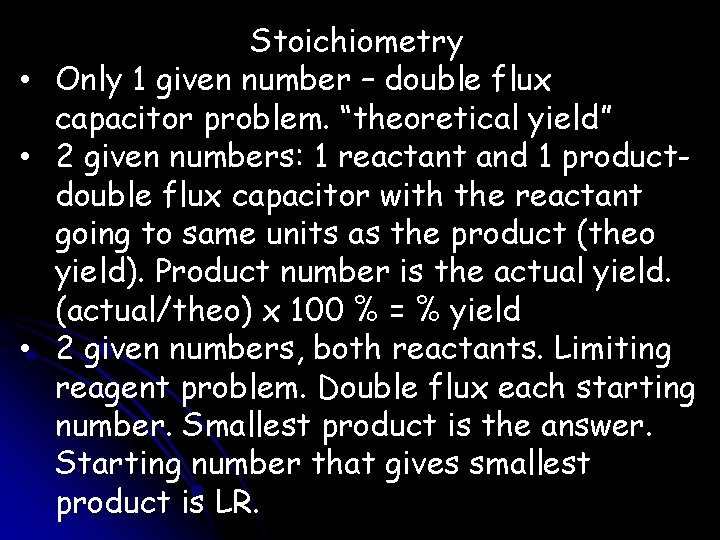Stoichiometry • Only 1 given number – double flux capacitor problem. “theoretical yield” • 2 given numbers: 1 reactant and 1 productdouble flux capacitor with the reactant going to same units as the product (theo yield). Product number is the actual yield. (actual/theo) x 100 % = % yield • 2 given numbers, both reactants. Limiting reagent problem. Double flux each starting number. Smallest product is the answer. Starting number that gives smallest product is LR.